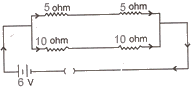Wednesday 03rd June 2020
 CBSE Guess > Papers > Question Papers > Class X > 2005 > Science > Delhi Set III SCIENCE AND TECHNOLOGY—2005 (Set III—Outside Delhi)
Except for the following questions, all the remaining questions have been asked in Set 1 and Set 2. SECTION A Q. 1. What is observed when a mixture of hydrogen and chlorine is exposed to sunlight? What is the product formed? 1 Q. 4. A wire of resistance 20 ohm is bent in the form of a closed circle. What is the effective resistance between the two points at the ends of any diameter of the circle? 1 Q. 7. Name the gas evolved when dilute sulphuric acid reacts with sodium carbonate. Write the chemical equation for the reaction involved. 2 Q. 10. Light enters from air into diamond which has a refractive index of 2.42. Calculate the speed of light in diamond. The speed of light in air is 3.0 x 108 ms-1. 2 Q. 13. Write the chemical equation representing the reaction in 'Haber's process' for the manufacture of ammonia gas. Mention for this process (i) the pressure, (ii) the temperature, (Hi) the catalyst and (iv) the promoter for the catalyst. 3 Q. 17. If a 6 V battery is connected to the arrangement of resistances given below, calculate: 3 (i) the total effective resistance of the arrangement and (ii) the total current flowing in the circuit.Q. 19. State Fleming's left-hand rule. With a labelled diagram, describe the working of an electric motor. What is the function of split ring commutator in a motor? 5 SECTION B Q. 21. What is a genetically modified organism (GMO)? 1 Q. 24. How do the following control the particulate emissions: 2 (i) Wet scrubbers (ii) Electrostatic precipitators. Q. 30. (a) Explain why the rate of photosynthesis in plants is low both at lower and higher temperatures? (b) Is green light most or least useful in photosynthesis and why? (c) Describe an activity to show that chloro phyll is necessary for photosynthesis in plants. 5 Science 2005 Question Papers Class X Delhi Outside Delhi Set I Set I Set II Set II Set III Set III CBSE 2005 Question Papers Class X